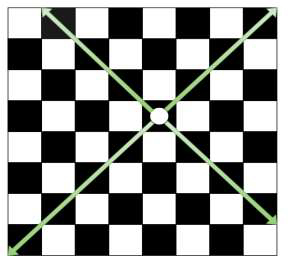# Count the total number of squares that can be visited by Bishop in one move in C++

On a chessboard represented as 8 X 8 grid we are given the position of Bishop in form of row and column position. The goal is to find the total number of squares that Bishop can visit in one move. We know the Bishop can move in all directions (diagonally left up/down and right up/down).For Example

## Input

row = 5, column = 4

## Output

Count of total number of squares that can be visited by Bishop in one move
are: 13

## Explanation

As shown in above figure the squares that Bishop can cover are 9.

## Input

row = 1, column = 1

## Output

Count of total number of squares that can be visited by Bishop in one move
are: 7

## Explanation

As this is the corner most position, then Bishop can only cover one
diagonal which can have a maximum of 7 squares.

Approach used in the below program is as follows

In this approach we will calculate the diagonal squares using horizontal and vertical maximum and minimum square position.

• Take integers row and column for position of bishop.

• Function squares_visited(int first, int second) takes the position of Bishop and returns the count of squares that it can visit in one move.

• Take the initial count as 0.

• The minimum of the left position is the minimum of row or column position −1.

• The maximum of the left position is 8 − maximum of row or 9−column position.

• The minimum of the right position is the minimum of row or 9−column position −1.

• The maximum of the right position is 8 − maximum of row or column position.

• Total squares will be the sum of above calculated positions.

• Return count as result.

## Example

Live Demo

#include <bits/stdc++.h>
using namespace std;
int squares_visited(int first, int second){
int count = 0;
int min_left = min(first, second) − 1;
int max_left = 8 − max(first, 9 − second);
int max_right = 8 − max(first, second);
int min_right = min(first, 9 − second) − 1;
count = min_left + min_right + max_right + max_left;
return count;
}
int main(){
int row = 3, column = 3;
cout<<"Count of total number of squares that can be visited by Bishop in one move are: "<<squares_visited(row, column);
return 0;
}

## Output

If we run the above code it will generate the following output −

Count of total number of squares that can be visited by Bishop in one move are: 11